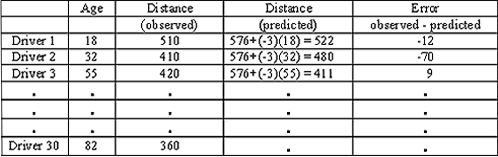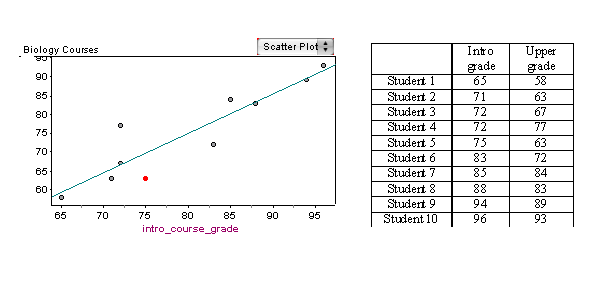## Use residuals, standard error, and r2 to assess the fit of a linear model.

### Learning Objectives

• Use residuals, standard error, and r2 to assess the fit of a linear model.

### Introduction

Let’s take a moment to summarize what we have done up to this point in Examining Relationships: Quantitative Data. Our goal from the beginning was to examine the relationship between two quantitative variables. We started by looking at scatterplots to see if we could see any pattern between the explanatory and response variables. We focused early in the course on identifying those cases that were linear in form. At the same time, we assessed how strong the linear relationship was on the basis of visual inspection. As is our usual strategy, we turned from graphs to numeric measures, and in particular, we developed the correlation coefficient, r, as a measure of the strength of the linear relationship we observed in the graph.

Once we established that there was a linear relationship between explanatory and response variables, the next step was to find a line that fit the data: the best-fit line. Here we used the least-squares method to find the regression line. Finally, we used the equation of the regression line to predict the value of the response variable for a given value of the explanatory variable.

### How Good Is the Best-Fit Line?

Now that we have a mathematical model (the least-squares regression line) that we can use to make predictions, we want to know: How good are these predictions, and how can we measure the error in a prediction?

## Highway Sign Visibility

Let’s begin our investigation by predicting the maximum distance that an 18-year-old driver can read a highway sign and then determining the error in our prediction.

We use the regression line equation:

Distance = 576 + (–3 * Age)

To predict the distance for an 18-year-old driver, we plug Age = 18 into the equation.

Predicted distance = 576 + (–3 * 18) = 522

Our prediction is that 522 feet is the maximum distance at which an 18-year-old driver can read a highway sign. Now let’s compare our prediction to the actual data for the 18-year-old driver: (18, 510).

The error in our prediction is 510 – 522 = –12.

This tells us that the actual distance for the 18-year-old driver is 12 feet closer than the prediction. In other words, our prediction is too large. It overestimates the actual distance by 12 feet.

So in general, we have Observed data value – Predicted value = Error.

If we use (x, y) to represent a typical data point and ŷ to represent the predicted value (obtained by using the regression equation), then we have

$\begin{array}{l}\text{observed}y-\text{predicted}y=\text{error}\\ y-ŷ=\text{error}\end{array}$

### Learn By Doing

Using this table showing “observed” and “predicted” distances for some drivers, find the following:https://assessments.lumenlearning.com/assessments/3497

Now let’s look at the error from a different perspective. We can think of the error as a way to adjust the prediction to match the data value.

From this point of view, we rewrite $y-ŷ=\text{error}$ as $y=ŷ+\text{error}$ .

This last equation says that the observed value is the predicted value plus the error. In other words, we can think of the error as the amount that we have to add to the prediction to get the observed value. From this point of view, the error can be thought of as a correction term. If the error is positive, it means the prediction is too small (the prediction underestimates the actual y-value). If the error is negative, it means the prediction is too large (the prediction overestimates the actual y-value).

The prediction error is also called a residual. So another way to express the previous equation is

$y=ŷ+\text{residual}$

In our next example, we look at prediction error from this point of view.

## Biology Courses

A biology department tracks the progress of students in its program. Grades in the introductory biology course have a strong linear relationship with grades in the upper-level biology courses (r = 0.91).

The least-squares regression equation isLet’s look at the predicted upper course grade for a student who makes a 75% in the introductory biology course.

Upper course grade = −8.9 + (1.05 * 75) = 69.85 ≈ 70

The regression line predicts that this student will make a 70% in the upper-level biology course.

The actual grade in the upper-level course for this student is 63%. The prediction is too high: it overestimates the data. To match the data value, we would need to subtract 7 from the prediction, so the error is −7.

In the scatterplot, notice that the regression line lies above the point (75, 63). Visually, we can see that the prediction is too high. This reinforces our previous observation that the prediction overestimates the data value. We would have to adjust the prediction downward to match the data value. Viewing the error as a correction term, we see the correction has to be negative.

Notice that when a point is close to the regression line, the prediction is close to the actual upper course grade, so the error is small. Another way to say this is that points close to the regression line have a small residual.